# 1st PUC Computer Science Model Question Paper 1 with Answers

Students can Download 1st PUC Computer Science Model Question Paper 1 with Answers, Karnataka 1st PUC Computer Science Model Question Papers with Answers helps you to revise the complete Karnataka State Board Syllabus and score more marks in your examinations.

## Karnataka 1st PUC Computer Science Model Question Paper 1 with Answers

Time: 3 Hrs 15 Min
Max. Marks: 100

PART – A

I. Answer all the following questions. Each question carries one mark. (10 × 1 = 10)

Question 1.
Who is called as the “Father of Computer”?
Charles Babbage is called as the “Father of Computer”.

Question 2.
Expand OMR?

Question 3.
Define debugging?
Bug is a problem in a program and debug means finding a solution to that problem.

Question 4.
Define a token?
The smallest individual units in a program are known as tokens.Question 5.
Which is the insertion operator?
cout is used in conjuction with << operator, known as insertion or put to operator.

Question 6.
What is a selection statement?
Selection statements are used to execute certain block of statements for evaluating the conditions.

Question 7.
What is the data type of the array subscript?
The data type of the array subscript is integer or int.

Question 8.
What does the keyword ‘void’ represent?
The data type ‘void’ has no values and no operations means it is empty. It plays a role of generic data type and can represent any of the other standard types.Question 9.
Which are the standard alignments used in a word processor?
The standard alignments used in a word processor are left, right, center and justify.

Question 10.
How many rows and columns are present in ESS?
There are 256 rows and 65536 columns in an electronic spread sheet.

PART – B

II. Answer any Five of the following questions. (5 × 2 = 10)

Question 11.
Mention any two features of ENIAC.
In one second, the ENIAC could perform 5,000 additions, 357 multiplications or 38 divisions.

Question 12.
List the different types of ROM?
The different types of ROM are Programmable ROM(PROM), Erasable Programmable ROM(EPROM) and Electrically Erasable Programmable ROM (EEPROM).Question 13.
What is an Operating system? Give an example.
An operating system or OS is a software program that enables the computer hardware to communicate and operate with the computer software. Windows 8 is an example of a latest operating system.

Question 14.
Write the advantages of structured programming?

1. Programs are easy to write because the programming logic is well organized.
2. Programs can be functionally divided into smaller logical working units (modularity).
3. Easy to maintain because of single entry and single exit.

Question 15.
Briefly explain any two characteristics of OOP?
Encapsulation and polymorphism are two characteristics of OOP.

1. Encapsulation:
The method of combining data, attributes, and methods in the same entity is called encapsulation.

2. Polymorphism:
Polymorphism is a Greek word which means many shapes. In OOP, Polymorphism means the ability to take on many forms. The term is applied both to objects and to operations.

Question 16.
Which are the different data types in C++?
The different data types in C++ are int, float, double and char.

Question 17.
What are the different types of values strcmp() can return?
The different values that a strcmp() function can return are zero, a positive number or a negative number.Question 18.
Give the commands for copy and paste?
The commands for copy and paste are Ctrl + c and Ctrl + v respectively.

PART – C

III. Answer any Five of the following questions. (5 × 3 = 15)

Question 19.
Explain impact and non-impact printers with examples?
1. Impact printers:
Impact printers forms characters or images by striking a mechanism such as a print hammer or wheel against an inked ribbon, leaving an image on the paper. For example dot matrix printer is an impact printer.

2. Non-impact printers:
Non-impact printers form characters and images without direct physical contact between the printing mechanism and the paper. For example ink jet printers is an non impact printer.

Question 20.
Subtract 36(10) from 83(10) using 2’s complement.
83(10) – 36(10)
Binary equivalent of 83(10) is 1010011(2)

 Divisor Dividend Remainder 2 83 1 2 41 1 2 20 0 2 10 0 2 5 1 2 2 0 2 1 1

Binary equivalent of 36(10) is 100100(2)

 Divisor Dividend Remainder 2 36 0 2 18 0 2 9 1 2 4 0 2 2 0 2 1 1

1 ’s complement of 0100100 is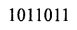2’s complement of 1011011 is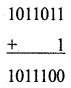Now, 83(10) 2’s complement of 36(10)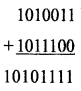A carry is generated and discarding it. The final answer is 101111(2)=47(10)Question 21.
What is meant by the terms multiprogramming and multitasking?
1. Multiprogramming:
Multiprogramming is the technique of running several programs at the same time using timesharing. It allows a computer to do several things simultaneously.

Running two or more programs at the same time on the same single-processor (single CPU) computer is called multitasking.

Question 22.
Write a flowchart to find the greater of two numbers?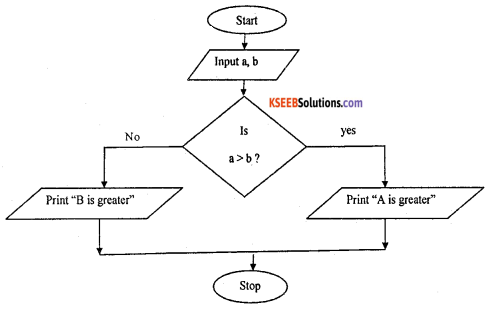Question 23.
Explain logical operators with example?
The operators which perform combine or negate the expressions that contain relational operators, are called logical operators.

Logical operators are used to combine one or more relational expressions. The logical operators are:

1. ! (NOT),
2. || (OR),
3. && (AND).

For example, if (age>18 && citizen =”Indian”)

Question 24.
Explain the cascading of input and output operators?
Cascading is a way to extract/insert multiple values from/into more than one variable using one cin/cout statement.
cout << “Value of B=” << b; Cascading of input operator (>>):
int n1, n2, n3;Question 25.
How do you initialize one dimensional array?
Initialization of one dimensional array:
int marks = {91, 96, 90, 94 ,99, 93};

Question 26.
Declare a structure that contains the data of a student?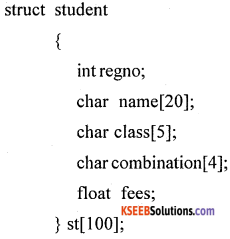PART – D

IV. Answer any Seven of the following questions. (7 × 5 = 35)

Question 27.
Explain the Generations of Computers in detail?
1. First-generation computers:The first computers used vacuum tubes for electronic circuitry and magnetic drums for memory. They were very expensive to operate and the power consumption was high, generated a lot of heat, which was often the cause of malfunctions.

2. Second-generation computers: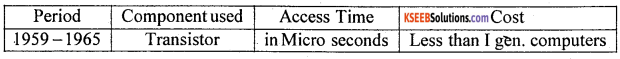In the second generation computers, symbolic or assembly languages were used. High-level programming languages were also in use at this time, such as early versions of COBOL and FORTRAN. Their memory consisted of magnetic core technology.

3. Third-generation computers: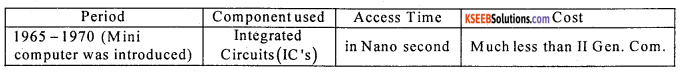The development of the integrated circuit was made use of the third generation computers. Transistors were miniaturized and were placed on silicon chips, called semiconductors.

• The processing speed and efficiency of computers increased.
• Keyboards and monitors were used as input and output devices.
• Computer system had an operating system and helped to run multiple applications simultaneously.

4. Fourth-generation computers: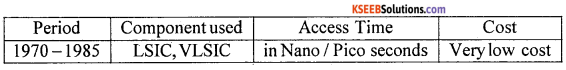The microprocessor was used in the fourth generation computers, as thousands of integrated _ circuits were built onto a single silicon chip. The Intel 4004 chip, developed in 1971, located all the components of the computer – from the central processing unit and memory to input/output controls – on a single chip.

Question 28.
FADE(16) = (7 5 3 3 6)(8)=(31454)(10)Question 29.
Explain ‘while’ structure with an example?
A ‘while’ loop statement repeatedly executes a statement or sequence of statements written in the flower brackets as long as a given condition returns the value ‘true’.
Syntax:
The syntax of a while loop in C++ is:
while(condition)
{
statement(s);
}
Here the condition may be any expression, and true for any non zero value. The loop iterates while the condition is true.
When the condition becomes false, program control passes to the line immediately following the loop.

During the first attempt, when the condition is tested and the result is false, the loop body will be skipped and the first statement after the while loop will be executed.

Example: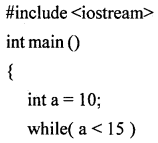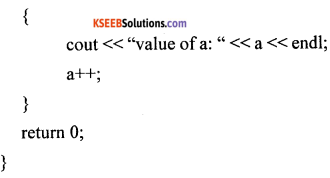When the above code is executed, it produces the following results:
value of a: 10
value of a: 11
value of a: 12
value of a: 13
value of a: 14

Question 30.
Explain the structure of C++ program. Give example?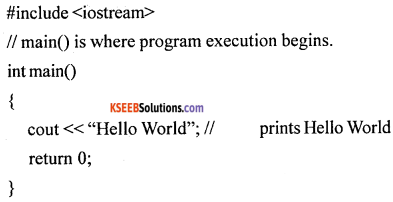The various parts of the above program:

• Headers, which contain information that is either necessary or useful to program. For this program, the header is needed.
• The next line // main() is where program execution begins, is a single-line comment available in C++. Single-line comments begin with // and stop at the end of the line.
• The line int main() is the main function where program execution begins.
• The pair of { } indicate the body of the main function.
• The next line cout << “Hello world.”; causes the message “Hello world” to be displayed on the screen.
• The next line return 0; terminates the main () function and causes it to return the value 0 to the calling process.

Question 31.
Explain the working of ‘for’ loop with an example
A ‘for’ loop is a repetition control structure that allows you to efficiently write a loop that needs to execute a specific number of times.
The syntax of a for loop in C++ is:
for (initialization; condition; increment/decrement)
{
statement(s);
}

The working of a ‘for’ loop:

1. The initialization step is executed first, and only once in the beginning. It is used to declare and initialize any loop control variables.

2. Next, the condition is evaluated. If it is true, the body of the loop is executed. If it is false, the body of the loop does not execute and flow of control jumps to the next statement just after the for loop.

3. After the body of the ‘for’ loop executes, the flow of control jumps back up to the increment/ decrement statement and updates any loop control variables.

4. Then condition is evaluated again. If it is true, the loop executes and the process repeats itself (body of loop, then increment step, and then again condition). After the condition becomes false, the for loop terminates.

Example: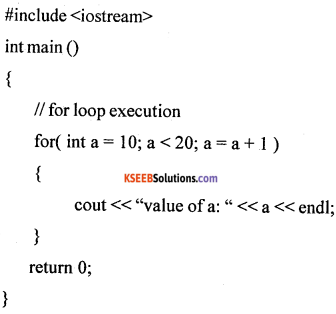When the above code is compiled and executed, it produces the following result:
value of a: 10
value of a: 11
value of a: 12
value of a: 13
value of a: 14
value of a: 15Question 32.
Write a C++ program to find the factorial of a given number?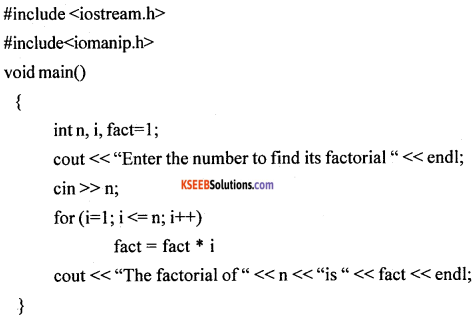Question 33.
Write a C++ program to read and print the elements of a two dimensional array.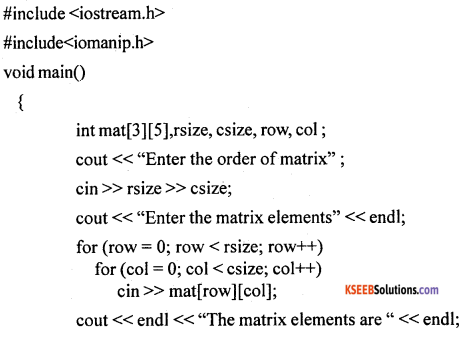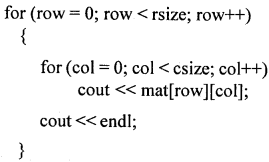Question 34.
Explain the working of “function with arguments and with no return value”? With an example.
Function with argument and no return value with an example:
The calling function main() gives the function call to the called function by passing arguments or values. Then the called function takes the values and performs the calculations and it self gives the output but no value sent back to the calling function.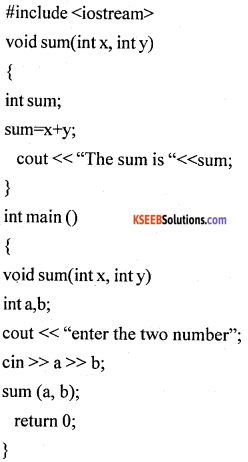In the above example, sum() is a user defined function. main() is a calling function which gives a function call sum (a, b); with actual arguments and The copy of values of a and b are sent to formal arguments x and y in the called function sum().

The function sum() performs addition and gives the result on the screen it can be deserved that. Here no value is sent back to the calling function main(). These kinds of functions are called “Function with arguments but no return values”.Question 35.
Explain any five built-in functions of ESS?
1. The sum():
It is used to find the addition for a range of cells. For example = sum(A1 : A20)

2. The product() :
It calculates the multiplication of values in the specified cells. For example, = product(B1:B5).

3. The max():
It finds the maximum element in the range of cells or sequence of values. For example, = max( 10, 20, 11, 45, 30) gives 45 as the output.

4. The min():
It finds the minimum element in the range of cells or sequence of values. For example, = min(10, 20, 11x, 45, 5, 30) gives 5 as the output.

5. The count():
It counts the number of elements present in the range of cells and sequence of values. For example, The Max (): It finds the maximum element in the range of cells or sequence of values.

Question 36.
Using ESS, analyze the result for the following data and represent it with the help of a Bar graph.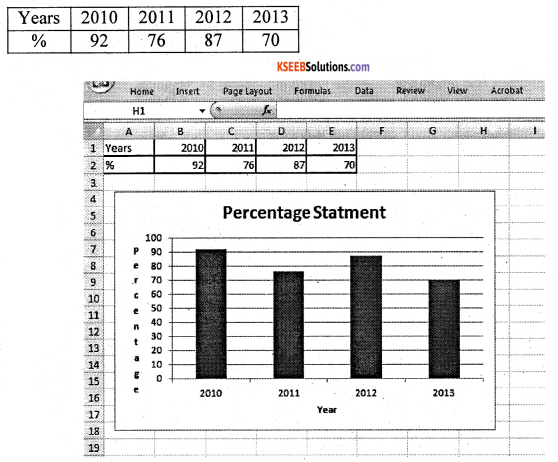From the above data and chart, it can be noted that in the year 2010 and 2012 percentage was higher compared to the years 2011 and 2013.

The year 2010 recorded the highest percentage about 92%, year 2012 recorded the second highest at 87% the third highest percentage was 76 for the year 2011 and year 2013 recorded the least percentage with 70%. To conclude, the performance is declining year by year which is alarming.Question 37.
Design a Web page to display your details on a website.
<HTML>
<BODY BGCOLOR=”Yellow”>
<CENTER> <H1> MY DETAILS
<IMG SRC = “C:\mydocuments\Pictures\cute%ganesha.jpg”>
</CENTER>
<FONT FACE =”algerian” size = “2” color = “blue”>
<OL>
<LI> NAME: GANESHA </LI>
<LI> FATHER’S NAME: SHIVA </LI>
<LI> MOTHER’S NAME: PARVATHI </LI>
<LI> ADDRESS: “ManasaGangothri,” 1st Cross, 1st Main, Himalayas. </LI>
<LI> HOBBIES: Reading, Painting, Dance </LI>
<LI> COLLEGE: Karnataka Pre-university Board </LI>
</OL>
</FONT>
<MARQUEE> Thanks for visiting my web page </MARQUEE>
</BODY>
</HTML>